# polaraxes

Create polar axes

## Syntax

``polaraxes``
``polaraxes(Name,Value)``
``polaraxes(parent,___)``
``pax = polaraxes(___)``
``polaraxes(pax_in)``

## Description

example

````polaraxes` creates the default polar axes in the current figure.```
````polaraxes(Name,Value)` specifies properties for the `PolarAxes` object using one or more name-value pair arguments, for example, `'ThetaDir','clockwise'`. For a list of properties, see PolarAxes Properties.```
````polaraxes(parent,___)` creates the polar axes in the figure, panel, or tab specified by `parent`, instead of in the current figure. Use this option alone or with name-value pair arguments.```
````pax = polaraxes(___)`returns the `PolarAxes` object created. Use `pax` to query and set properties of the `PolarAxes` object after it is created. For a list of properties, see PolarAxes Properties.```

example

````polaraxes(pax_in)` makes the `PolarAxes` object `pax_in` the current axes.```

## Examples

collapse all

Create a new figure with polar axes and assign the polar axes object to `pax`. Add a plot to the axes. Then, use `pax` to modify axes properties.

```figure pax = polaraxes; theta = 0:0.01:2*pi; rho = sin(2*theta).*cos(2*theta); polarplot(theta,rho) pax.ThetaDir = 'clockwise'; pax.FontSize = 12;```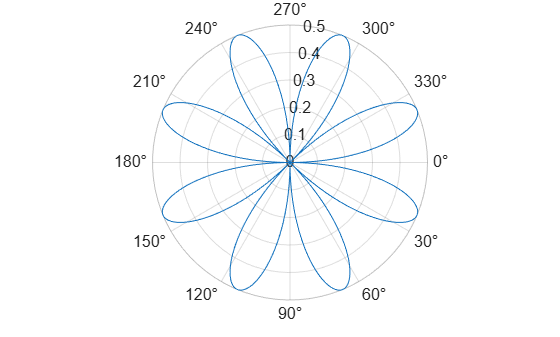Before R2022a, polar axes do not include degree symbols by default. To add them, get the polar axes using `pax = gca`. Then modify the tick labels using `pax.ThetaTickLabel = string(pax.ThetaTickLabel) + char(176)`.

Create a figure with polar axes and assign the polar axes object to `pax`. Then, ensure `pax` is the current axes before calling the `polarplot` function.

```figure pax = polaraxes; polaraxes(pax) polarplot(1:10)```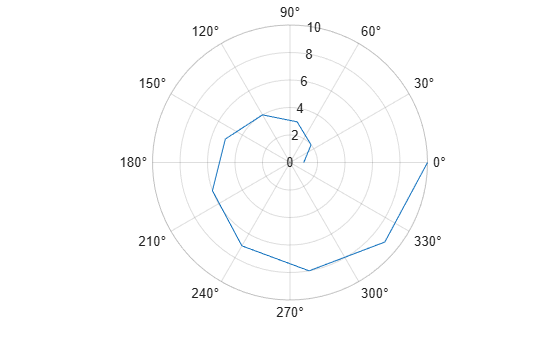## Input Arguments

collapse all

Parent container, specified as a `Figure`, `Panel`, `Tab`, `TiledChartLayout`, or `GridLayout` object.

Polar axes to make current, specified as a `PolarAxes` object.

### Name-Value Arguments

Specify optional pairs of arguments as `Name1=Value1,...,NameN=ValueN`, where `Name` is the argument name and `Value` is the corresponding value. Name-value arguments must appear after other arguments, but the order of the pairs does not matter.

Before R2021a, use commas to separate each name and value, and enclose `Name` in quotes.

Example: `'ThetaZeroLocation','top','ThetaDir','clockwise'`

The properties listed here are only a subset. For a complete list, see PolarAxes Properties. Some graphics functions reset axes properties when plotting. To avoid graphics functions from overriding the property values, set axes properties after plotting.

Units for angle values, specified as one of these values:

• `'degrees'` — Label the angles in degrees, and interpret the `ThetaLim`, `ThetaTick`, and `RAxisLocation` property values in degrees. When you switch the units from radians to degrees, MATLAB® converts the radian values in those three properties to the equivalent degree values.

• `'radians'` — Label the angles in radians, and interpret the `ThetaLim`, `ThetaTick`, and `RAxisLocation` property values in radians. When you switch the units from degrees to radians, MATLAB converts the degree values in those three properties to the equivalent radian values.

Example: `ax.ThetaAxisUnits = 'radians';`

Location of the zero reference axis, specified as one of the values in this table.

ValueResult
`'right'`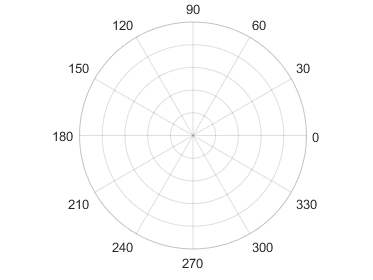`'top'`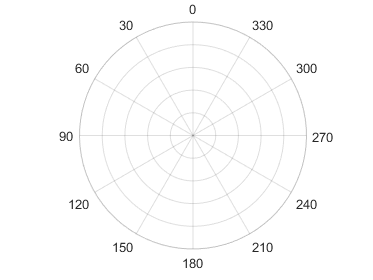`'left'`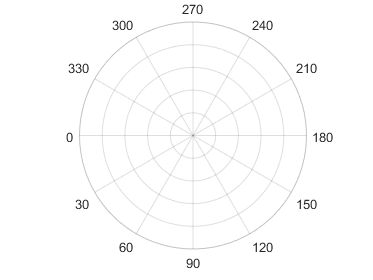`'bottom'`Example: `ax.ThetaZeroLocation = 'left';`

Direction of increasing angles, specified as one of the values in this table.

ValueResult
`'counterclockwise'`

Angles increase in a counterclockwise direction.`'clockwise'`

Angles increase in a clockwise direction.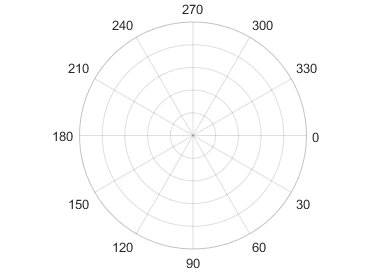Example: `ax.ThetaDir = 'clockwise';`

Minimum and maximum angle values, specified as a two-element vector of the form `[thmin thmax]`. If the difference between the values is less than 360 degrees, then the theta-axis is a partial circle.

MATLAB interprets the values in units determined by the `ThetaAxisUnits` property.

Example: `ax.ThetaLim = [0 180];`

Angles at which to display lines extending from the origin, specified as a vector of increasing values. MATLAB labels the lines with the appropriate angle values, unless you specify different labels using the `ThetaTickLabel` property.

MATLAB interprets the values in units determined by the `ThetaAxisUnits` property.

Example: `ax.ThetaTick = [0 90 180 270];`

Alternatively, specify the values using the `thetaticks` function.

Color of the theta-axis, including the theta-axis grid lines, tick marks, tick labels. Specify this value as an RGB triplet, a hexadecimal color code, a color name, or a short name.

Note

If you specify the `GridColor` property, then the grid lines use the color in the `GridColor` property instead. See `GridColorMode` for more information.

For a custom color, specify an RGB triplet or a hexadecimal color code.

• An RGB triplet is a three-element row vector whose elements specify the intensities of the red, green, and blue components of the color. The intensities must be in the range `[0,1]`, for example, ```[0.4 0.6 0.7]```.

• A hexadecimal color code is a string scalar or character vector that starts with a hash symbol (`#`) followed by three or six hexadecimal digits, which can range from `0` to `F`. The values are not case sensitive. Therefore, the color codes `"#FF8800"`, `"#ff8800"`, `"#F80"`, and `"#f80"` are equivalent.

Alternatively, you can specify some common colors by name. This table lists the named color options, the equivalent RGB triplets, and hexadecimal color codes.

Color NameShort NameRGB TripletHexadecimal Color CodeAppearance
`"red"``"r"``[1 0 0]``"#FF0000"``"green"``"g"``[0 1 0]``"#00FF00"``"blue"``"b"``[0 0 1]``"#0000FF"``"cyan"` `"c"``[0 1 1]``"#00FFFF"``"magenta"``"m"``[1 0 1]``"#FF00FF"``"yellow"``"y"``[1 1 0]``"#FFFF00"``"black"``"k"``[0 0 0]``"#000000"``"white"``"w"``[1 1 1]``"#FFFFFF"``"none"`Not applicableNot applicableNot applicableNo color

Here are the RGB triplets and hexadecimal color codes for the default colors MATLAB uses in many types of plots.

`[0 0.4470 0.7410]``"#0072BD"``[0.8500 0.3250 0.0980]``"#D95319"``[0.9290 0.6940 0.1250]``"#EDB120"``[0.4940 0.1840 0.5560]``"#7E2F8E"``[0.4660 0.6740 0.1880]``"#77AC30"``[0.3010 0.7450 0.9330]``"#4DBEEE"``[0.6350 0.0780 0.1840]``"#A2142F"`For example, `ax.ThetaColor = 'r'` changes the color to red.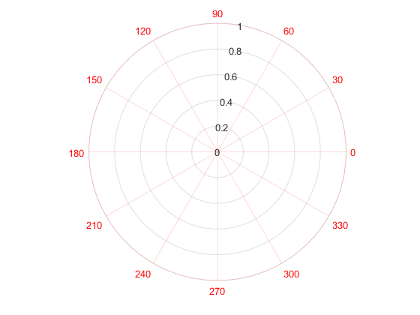Location of the r-axis, specified a scalar angle value. MATLAB interprets the values in units determined by the `ThetaAxisUnits` property.

Example: `ax.RAxisLocation = 90;`

Direction of increasing values along the r-axis, specified as one of these values:

• `'normal'` — Values increase outward from the center of the chart. The radius at the origin corresponds to the minimum value stored in the `RLim` property (typically 0).

• `'reverse'` — Values decrease outward from the center of the chart. The radius at the origin corresponds to the maximum value stored in the `RLim` property.

Example: `ax.RDir = 'reverse';`

Minimum and maximum radius limits, specified as a two-element vector of the form `[rmin rmax]`, where `rmax` is a numeric value greater than `rmin`. You can specify both limits, or specify one limit and let the axes automatically calculate the other.

• To automatically set the minimum limit to the minimum data value, specify the first element as `-inf`, for example, `[-inf 0]`.

• To automatically set the maximum limit to the maximum data value, specify the second element as `inf`, for example, `[0 inf]`.

Alternatively, use the `rlim` function to set the limits.

Example: `ax.RLim = [0 6];`

Radius tick values, specified as a vector of increasing values. The radius tick values are the locations along the r-axis where the circular lines appear. The radius tick labels are the labels that you see next to each line. Use the `RTickLabels` property to specify the associated labels.

Example: `ax.RTick = [0 2 4 6];`

Alternatively, use the `rticks` function to specify the tick values.

Color of the r-axis, including the r-axis grid lines, tick marks, and tick labels. Specify this value as an RGB triplet, a hexadecimal color code, a color name, or a short name.

Note

If you specify the `GridColor` property, then the grid lines use the color in the `GridColor` property instead. See `GridColorMode` for more information.

For a custom color, specify an RGB triplet or a hexadecimal color code.

• An RGB triplet is a three-element row vector whose elements specify the intensities of the red, green, and blue components of the color. The intensities must be in the range `[0,1]`, for example, ```[0.4 0.6 0.7]```.

• A hexadecimal color code is a string scalar or character vector that starts with a hash symbol (`#`) followed by three or six hexadecimal digits, which can range from `0` to `F`. The values are not case sensitive. Therefore, the color codes `"#FF8800"`, `"#ff8800"`, `"#F80"`, and `"#f80"` are equivalent.

Alternatively, you can specify some common colors by name. This table lists the named color options, the equivalent RGB triplets, and hexadecimal color codes.

Color NameShort NameRGB TripletHexadecimal Color CodeAppearance
`"red"``"r"``[1 0 0]``"#FF0000"``"green"``"g"``[0 1 0]``"#00FF00"``"blue"``"b"``[0 0 1]``"#0000FF"``"cyan"` `"c"``[0 1 1]``"#00FFFF"``"magenta"``"m"``[1 0 1]``"#FF00FF"``"yellow"``"y"``[1 1 0]``"#FFFF00"``"black"``"k"``[0 0 0]``"#000000"``"white"``"w"``[1 1 1]``"#FFFFFF"``"none"`Not applicableNot applicableNot applicableNo color

Here are the RGB triplets and hexadecimal color codes for the default colors MATLAB uses in many types of plots.

`[0 0.4470 0.7410]``"#0072BD"``[0.8500 0.3250 0.0980]``"#D95319"``[0.9290 0.6940 0.1250]``"#EDB120"``[0.4940 0.1840 0.5560]``"#7E2F8E"``[0.4660 0.6740 0.1880]``"#77AC30"``[0.3010 0.7450 0.9330]``"#4DBEEE"``[0.6350 0.0780 0.1840]``"#A2142F"`For example, `ax.RColor = 'r'` changes the color to red.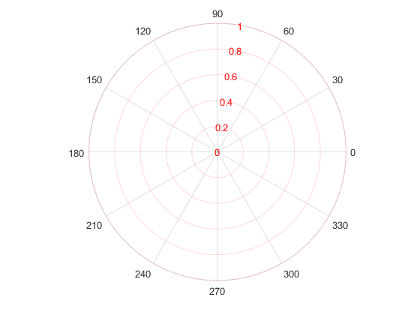## Version History

Introduced in R2016a

expand all